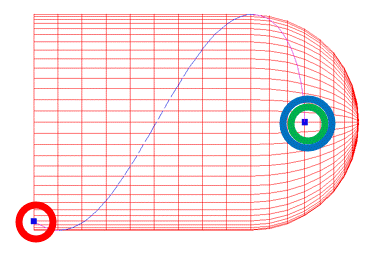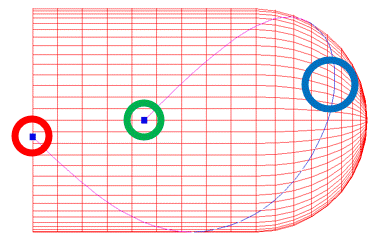# Cadfil Example of a FIB path with a symmetric mandrel

### Symmetric Mandrel X- (Left) Side

This is an example of a symmetric mandrel, note the mandrel has only three points which define the right hand side (half) of the mandrel. The left side is created automatically because the X- end symmetry button is selected.With a symmetric mandrel from the mandrel editor a complete path needs only one turning point, the second turning point is on the other (virtual) dome and is created by the symmetry. The symmetric path must touch the symmetry plane so the start or end point must be on the symmetry plane. Below are three valid examples of fibre paths. Because in all cases the first turning point is on the right side dome this is where the program start position will be. If you wanted to start on the left side dome the other symmetric mandrel case (X+) should have been set.

### Key to the 3 example figures### Example 1: Start X=250, Angle = Hoop (+90)### Example 2: Start X=0, Angle = +45### Example 3: Start X=100, Angle = +45### Payout path has full mandrel

When the payout path is created from the symmetric half path the full part with both sides is made and the two sides should be exactly the same.Updated: